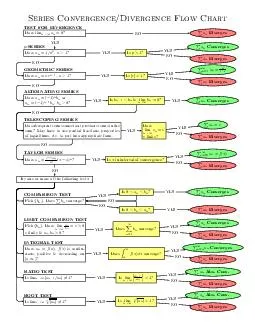# Series ConvergenceDivergence Flow Chart TEST FOR DIVERGENCE Does lim Diverges NO SERIES Does n YES Is p YES Converges YES Diverges NO GEOMETRIC SERIES Does ar NO Is YES YES Diverges NO ALTEEmbed code:

## Series ConvergenceDivergence Flow Chart TEST FOR DIVERGENCE Does lim Diverges NO SERIES Does n YES Is p YES Converges YES Diverges NO GEOMETRIC SERIES Does ar NO Is YES YES Diverges NO ALTE

Download Pdf - The PPT/PDF document "Series ConvergenceDivergence Flow Chart ..." is the property of its rightful owner. Permission is granted to download and print the materials on this web site for personal, non-commercial use only, and to display it on your personal computer provided you do not modify the materials and that you retain all copyright notices contained in the materials. By downloading content from our website, you accept the terms of this agreement.

### Presentations text content in Series ConvergenceDivergence Flow Chart TEST FOR DIVERGENCE Does lim Diverges NO SERIES Does n YES Is p YES Converges YES Diverges NO GEOMETRIC SERIES Does ar NO Is YES YES Diverges NO ALTE

Page 1
Series Convergence/Divergence Flow Chart TEST FOR DIVERGENCE Does lim = 0? Diverges NO -SERIES Does = 1 /n 1? YES Is p > 1? YES Converges YES Diverges NO GEOMETRIC SERIES Does ar 1? NO Is 1? YES =1 YES Diverges NO ALTERNATING SERIES Does = ( 1) or = ( 1) 0? NO Is +1 & lim = 0? YES Converges YES TELESCOPING SERIES Do subsequent terms cancel out previous terms in the sum? May have to use partial fractions, properties of logarithms, etc. to put into appropriate form. NO Does lim ﬁnite? YES YES Diverges NO TAYLOR SERIES Does NO Is in interval of convergence? YES =0 YES

Diverges NO Try one or more of the following tests: NO COMPARISON TEST Pick . Does converge? Is 0 YES Converges YES Is 0 NO NO Diverges YES LIMIT COMPARISON TEST Pick . Does lim c > ﬁnite & , b 0? Does =1 converge? YES Converges YES Diverges NO INTEGRAL TEST Does ), ) is contin- uous, positive & decreasing on a, )? Does dx converge? YES Converges YES Diverges NO RATIO TEST Is lim +1 /a |6 = 1? Is lim +1 1? YES Abs. Conv. YES Diverges NO ROOT TEST Is lim = 1? Is lim 1? YES Abs. Conv. YES Diverges NO
Page 2
Problems 1-38 from Stewart’s Calculus , page 784 1. =1 2. =1 3. =1 4. =1

1) 5. =1 3) +1 6. =1 1 + 8 7. =2 ln( 8. =1 + 2)! 9. =1 10. =1 11. =2 1) +1 ln( 12. =1 1) + 25 13. =1 14. =1 sin( 15. =0 (3 + 2) 16. =1 + 1 + 1 17. =1 1) /n 18. =2 1) 19. =1 1) ln( 20. =1 + 5 21. =1 2) 22. =1 + 2 + 5 23. =1 tan(1 /n 24. =1 cos( n/ 2) + 4 25. =1 26. =1 + 1 27. =1 ln( + 1) 28. =1 /n 29. =1 tan 30. =1 1) + 5 31. =1 + 4 32. =1 (2 33. =1 sin(1 /n 34. =1 cos 35. =1 + 1 36. =2 (ln( )) ln( 37. =1 1) 38. =1 1)